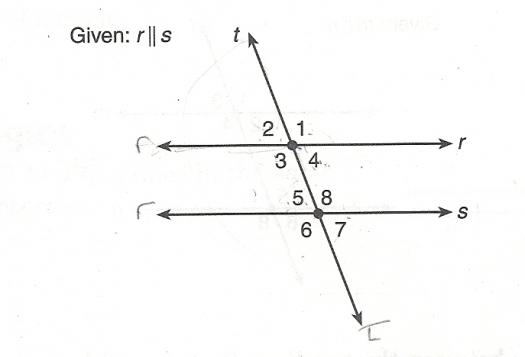# Unit 2 - 7th Grade Quiz One

9 Questions | Total Attempts: 80SettingsTests whether student is able to identify angles created by two parallel lines and a transversal, and on the Triangle Inequality Thereom. Use the following diagram to answer questions 1 through 9.

Related Topics
• 1.
Which angles represent corresponding angles?
• A.

• B.

• C.

• D.

• 2.
Which angles represent alternate exterior angles?
• A.

• B.

• C.

• D.

• 3.
Which angles represent alternate interior angles?
• A.

• B.

• C.

• D.

• 4.
Which angles are supplementary?
• A.

• B.

• C.

• D.

>6 and

• 5.
If m
• A.

20 degrees

• B.

110 degrees

• C.

70 degrees

• D.

Can't tell

• 6.
If m
• A.

110 degrees

• B.

70 degrees

• C.

180 degrees

• D.

Can't tell

• 7.
Which angle is NOT an interior angle?
• A.

• B.

• C.

• D.

• 8.
Determine whether the given side lengths can form a triangle.4in., 9in., 12 in.
• A.

Yes

• B.

No

• 9.
Determine whether the given side lengths can form a triangle.2 cm, 18 cm, 21 cm.
• A.

Yes

• B.

No